• hdsht函数的和、差、积、商的求导法则 二、复合函数的求导法则 三、高阶导函数的和、差、积、商的求导法则 二、复合函数的求导法则 三、高阶导数数reyefgfdgggggggggggggggggggg
• 目前来说，我在市面上还没找到对于高维Tensor求导法则的详细介绍。比如说推导CNN的时候，必须用kronecker product来回折腾。对于RNN，则干脆就求不出来。这里介绍一个通用的资源数学
• ## 矩阵求导法则与性质

万次阅读 多人点赞 2018-07-04 16:04:52
介绍矩阵求导法则，以及常用的求导公式、迹函数、行列式求导结论 矩阵求导法则 矩阵求导应该分为标量求导、向量求导、矩阵求导三个方面来介绍，公式繁多，但仔细看看其实是有规律可循的。 标量求导 无论是...
介绍矩阵求导法则，以及常用的求导公式、迹函数、行列式求导结论

矩阵求导法则
矩阵求导应该分为标量求导、向量求导、矩阵求导三个方面来介绍，公式繁多，但仔细看看其实是有规律可循的。
标量求导
无论是矩阵、向量对标量求导，或者是标量对矩阵、向量求导，其结论都是一样的：等价于对矩阵（向量）的每个分量求导，并且保持维数不变。
例如，我们可以计算标量对向量求导：
设

y

y

$y$为一个元素，xT=[x1...xq]${x}^{T}=\left[{x}_{1}...{x}_{q}\right]$$x^T = [x_1...x_q]$是

q

q

$q$维行向量，则：

∂y∂xT=[∂y∂x1...∂y∂xq]$\frac{\mathrm{\partial }y}{\mathrm{\partial }{x}^{T}}=\left[\frac{\mathrm{\partial }y}{\mathrm{\partial }{x}_{1}}...\frac{\mathrm{\partial }y}{\mathrm{\partial }{x}_{q}}\right]$\frac{\partial y}{\partial x^T} = [\frac{\partial y}{\partial x_1}...\frac{\partial y}{\partial x_q}]
向量求导
对于向量求导，我们可以先将向量看做一个标量，然后使用标量求导法则，最后将向量形式化为标量进行。
例如，我们可以计算行向量对列向量求导：
设

yT=[y1...yn]

y

T

=

[

y

1

.

.

.

y

n

]

$y^T=[y_1...y_n]$是

n

n

$n$维行向量，x=[x1,...,xp]$x=\left[{x}_{1},...,{x}_{p}\right]$$x=[x_1,...,x_p]$是

p

p

$p$维列向量，则：

∂yT∂x==[∂y1∂x...∂yn∂x]⎡⎣⎢⎢⎢∂y1∂x1...∂y1∂xp.........∂yn∂x1...∂yn∂xp⎤⎦⎥⎥⎥$\begin{array}{rlr}\frac{\mathrm{\partial }{y}^{T}}{\mathrm{\partial }x}& =& \left[\frac{\mathrm{\partial }{y}_{1}}{\mathrm{\partial }x}...\frac{\mathrm{\partial }{y}_{n}}{\mathrm{\partial }x}\right]\\ & =& \left[\begin{array}{ccc}\frac{\mathrm{\partial }{y}_{1}}{\mathrm{\partial }{x}_{1}}& ...& \frac{\mathrm{\partial }{y}_{n}}{\mathrm{\partial }{x}_{1}}\\ ...& ...& ...\\ \frac{\mathrm{\partial }{y}_{1}}{\mathrm{\partial }{x}_{p}}& ...& \frac{\mathrm{\partial }{y}_{n}}{\mathrm{\partial }{x}_{p}}\end{array}\right]\end{array}$\begin{align}\frac{\partial y^T}{\partial x}  &=& [\frac{\partial y_1}{\partial x}...\frac{\partial y_n}{\partial x}] \nonumber  \\ &=&\begin{bmatrix}\frac{\partial y_1}{\partial x_1}& ... &\frac{\partial y_n}{\partial x_1} \\ ... & ... &... \\\frac{\partial y_1}{\partial x_p} &...  &\frac{\partial y_n}{\partial x_p} \end{bmatrix} \nonumber\end{align}
矩阵求导
与向量求导类似，先将矩阵化当做一个标量，再使用标量对矩阵的运算进行。
例如，我们可以计算矩阵对列向量求导：
设

Y=⎡⎣⎢y11...ym1.........y1n...ymn⎤⎦⎥

Y

=

[

y

11

.

.

.

y

1

n

.

.

.

.

.

.

.

.

.

y

m

1

.

.

.

y

m

n

]

$Y =\begin{bmatrix}y_{11} &... & y_{1n} \\... &... &... \\ y_{m1} & ...&y_{mn} \end{bmatrix}$是

m×n

m

×

n

$m\times n$矩阵，

x=[x1,...,xp]

x

=

[

x

1

,

.

.

.

,

x

p

]

$x=[x_1,...,x_p]$是

p

p

$p$维列向量，则：

∂Y∂x=[∂Y∂x1,...,∂Y∂xp]$\frac{\mathrm{\partial }Y}{\mathrm{\partial }x}=\left[\frac{\mathrm{\partial }Y}{\mathrm{\partial }{x}_{1}},...,\frac{\mathrm{\partial }Y}{\mathrm{\partial }{x}_{p}}\right]$\frac{\partial Y}{\partial x}  = [\frac{\partial Y}{\partial x_1},...,\frac{\partial Y}{\partial x_p}]
矩阵微积分
常见求导性质
实值函数相对于实向量的梯度
设

f(x)=x=[x1,...,xn]T

f

(

x

)

=

x

=

[

x

1

,

.

.

.

,

x

n

]

T

$f(x) = x = [x_1,...,x_n]^T$

∂f(x)∂xT=∂x∂xT=In×n

∂

f

(

x

)

∂

x

T

=

∂

x

∂

x

T

=

I

n

×

n

\frac{\partial f (x)}{\partial x^T}  = \frac{\partial x}{\partial x^T}  = I_{n\times n}

∂(f(x))T∂x=∂xT∂x=In×n

∂

(

f

(

x

)

)

T

∂

x

=

∂

x

T

∂

x

=

I

n

×

n

\frac{\partial (f (x))^T}{\partial x}  = \frac{\partial x^T}{\partial x}  = I_{n\times n}

∂f(x)∂x=∂x∂x=vec(In×n)

∂

f

(

x

)

∂

x

=

∂

x

∂

x

=

v

e

c

(

I

n

×

n

)

\frac{\partial f (x)}{\partial x}  = \frac{\partial x}{\partial x}  = vec(I_{n\times n})

∂(f(x))T∂xT=∂xT∂xT=vec(In×n)T

∂

(

f

(

x

)

)

T

∂

x

T

=

∂

x

T

∂

x

T

=

v

e

c

(

I

n

×

n

)

T

\frac{\partial (f (x))^T}{\partial x^T}  = \frac{\partial x^T}{\partial x^T}  = vec(I_{n\times n})^T

其中，

vec

v

e

c

$vec$表示向量化矩阵，按列将矩阵表示为向量，具体可见Wikipedia。
常见性质

f(x)=Ax

f

(

x

)

=

A

x

$f(x) = Ax$，则

∂f(x)∂xT=∂(Ax)∂xT=A

∂

f

(

x

)

∂

x

T

=

∂

(

A

x

)

∂

x

T

=

A

\frac{\partial f (x)}{\partial x^T}  = \frac{\partial (Ax)}{\partial x^T}  =A

f(x)=xTAx

f

(

x

)

=

x

T

A

x

$f(x) = x^TAx$，则

∂f(x)∂x=∂(xTAx)∂x=Ax+ATx

∂

f

(

x

)

∂

x

=

∂

(

x

T

A

x

)

∂

x

=

A

x

+

A

T

x

\frac{\partial f (x)}{\partial x}  = \frac{\partial (x^TAx)}{\partial x}  =Ax+A^Tx

f(x)=aTx

f

(

x

)

=

a

T

x

$f(x) = a^Tx$，则

∂aTx∂x=∂xTa∂x=a

∂

a

T

x

∂

x

=

∂

x

T

a

∂

x

=

a

\frac{\partial a^Tx}{\partial x}  = \frac{\partial x^Ta}{\partial x}  =a

f(x)=xTAy

f

(

x

)

=

x

T

A

y

$f(x) = x^TAy$，则

∂xTAy∂x=Ay

∂

x

T

A

y

∂

x

=

A

y

\frac{\partial x^TAy}{\partial x}  = Ay

∂xTAy∂A=xyT

∂

x

T

A

y

∂

A

=

x

y

T

\frac{\partial x^TAy}{\partial A}  = xy^T

df(X)=tr((∂f(X)∂X)TdX)

d

f

(

X

)

=

t

r

(

(

∂

f

(

X

)

∂

X

)

T

d

X

)

df(X) = tr((\frac{\partial f(X)}{\partial X})^T d X)矩阵微分也满足线性法则、乘积法则。矩阵的逆的微分

d(X−1)=−X−1(dX)X−1

d

(

X

−

1

)

=

−

X

−

1

(

d

X

)

X

−

1

d(X^{-1}) = -X^{-1}(dX)X^{-1}
迹函数
迹函数相对于矩阵的梯度

∂(tr(ZZT))∂Z=∂(tr(ZTZ))∂Z=2Z

∂

(

t

r

(

Z

Z

T

)

)

∂

Z

=

∂

(

t

r

(

Z

T

Z

)

)

∂

Z

=

2

Z

\frac{\partial (tr (ZZ^T))}{\partial Z}  = \frac{\partial (tr (Z^TZ))}{\partial Z}  = 2Z

矩阵微分算子和迹算子的可交换性

d(tr(X))=tr(d(X))=∑i=1ndxii

d

(

t

r

(

X

)

)

=

t

r

(

d

(

X

)

)

=

∑

i

=

1

n

d

x

i

i

d(tr(X)) = tr(d(X)) = \sum\limits_{i=1}^{n} dx_{ii}

常见性质

∂tr(A)∂A=In×n

∂

t

r

(

A

)

∂

A

=

I

n

×

n

\frac{\partial tr(A)}{\partial A}  = I_{n\times n}

∂tr(AB)∂A=BT

∂

t

r

(

A

B

)

∂

A

=

B

T

\frac{\partial tr(AB)}{\partial A}  = B^T

d(tr(AXB))=tr(A(dX)B)=tr(BA(dx))

d

(

t

r

(

A

X

B

)

)

=

t

r

(

A

(

d

X

)

B

)

=

t

r

(

B

A

(

d

x

)

)

d(tr(AXB)) = tr(A(dX)B) = tr(BA(dx))

∂tr(AXB)∂X=(BA)T=ATBT

∂

t

r

(

A

X

B

)

∂

X

=

(

B

A

)

T

=

A

T

B

T

\frac{\partial tr(AXB)}{\partial X}  = (BA)^T = A^TB^T

d(tr(AX−1B))=tr(A(dX−1)B)=−tr(AX−1(dX)X−1B)=−tr(X−1BAX−1dX)

d

(

t

r

(

A

X

−

1

B

)

)

=

t

r

(

A

(

d

X

−

1

)

B

)

=

−

t

r

(

A

X

−

1

(

d

X

)

X

−

1

B

)

=

−

t

r

(

X

−

1

B

A

X

−

1

d

X

)

d(tr(AX^{-1}B)) = tr(A(dX^{-1})B) = -tr(AX^{-1}(dX)X^{-1}B) = -tr(X^{-1}BAX^{-1}dX)

∂tr(AX−1B)∂X=−(X−1BAX−1)T=−X−TATBTX−T

∂

t

r

(

A

X

−

1

B

)

∂

X

=

−

(

X

−

1

B

A

X

−

1

)

T

=

−

X

−

T

A

T

B

T

X

−

T

\frac{\partial tr(AX^{-1}B)}{\partial X} = -(X^{-1}BAX^{-1})^T = -X^{-T}A^TB^TX^{-T}

∂tr(XTX)∂X=2X

∂

t

r

(

X

T

X

)

∂

X

=

2

X

\frac{\partial tr(X^TX)}{\partial X}  = 2X ​
行列式
行列式相对于矩阵的梯度

∂|Z|∂Z=|Z|(Z−1)T

∂

|

Z

|

∂

Z

=

|

Z

|

(

Z

−

1

)

T

\frac{\partial |Z|}{\partial Z}   = |Z|(Z^{-1})^T

微分形式

d|X|=tr(|X|X−1dX)

d

|

X

|

=

t

r

(

|

X

|

X

−

1

d

X

)

d|X| = tr(|X| X^{-1} dX)

常见性质

d|AXB|===tr(|AXB|(AXB)−1d(AXB))tr(|AXB|(AXB)−1A(dX)B)tr(|AXB|B(AXB)−1A(dX))

d

|

A

X

B

|

=

t

r

(

|

A

X

B

|

(

A

X

B

)

−

1

d

(

A

X

B

)

)

=

t

r

(

|

A

X

B

|

(

A

X

B

)

−

1

A

(

d

X

)

B

)

=

t

r

(

|

A

X

B

|

B

(

A

X

B

)

−

1

A

(

d

X

)

)

\begin{align} d|AXB| &=& tr(|AXB| (AXB)^{-1}d(AXB)) \nonumber\\&=& tr(|AXB| (AXB)^{-1}A(dX)B) \nonumber\\&=& tr(|AXB| B(AXB)^{-1}A(dX))\nonumber\end{align}

∂|AXB|∂X=|AXB|AT(BTXTAT)−1BT

∂

|

A

X

B

|

∂

X

=

|

A

X

B

|

A

T

(

B

T

X

T

A

T

)

−

1

B

T

\frac{\partial |AXB|}{\partial X}   = |AXB|A^T(B^TX^TA^T)^{-1}B^T

∂|X|∂X=|X|X−T

∂

|

X

|

∂

X

=

|

X

|

X

−

T

\frac{\partial |X|}{\partial X} = |X| X^{-T}

∂|XXT|∂X=2|XXT|(XXT)−1X

∂

|

X

X

T

|

∂

X

=

2

|

X

X

T

|

(

X

X

T

)

−

1

X

\frac{\partial |XX^T|}{\partial X} = 2|XX^T| (XX^{T})^{-1}X
reference
矩阵的导数与迹
展开全文• 本篇内容为四则和复合的求导法则和证明 第二部分 求导法则（四则、复合、反函数求导） 四则求导法则 加减法，以加法为例 乘法 除法 复合求导法则 反函数求导法则 y=f(x)要严格单调才有反函数 反函数解法：y=f...
本篇内容为四则和复合的求导法则和证明
第二部分 求导法则（四则、复合、反函数求导）
四则求导法则加减法，以加法为例乘法除法复合求导法则反函数求导法则 y=f(x)要严格单调才有反函数 反函数解法：y=f(x)→x=f(y)，调换x,y，这是中学的解法，高等数学中将y=f(x)解成x=f(y)的形式，不做x,y的调换,也就是说，x还是原来的x，y还是原来的y总结
本篇内容为求导法则（四则、复合、反函数）的证明，为下一篇总结常用的求导公式做铺垫，在下一届中，在求导公式推导中练习本篇的理论。
预：常用求导公式总结及推导
展开全文• 一、函数的和差、积商的求导法则 1.1、定理1 1.2、证明，通过极限证明 二、反函数的求导法则 2.1、定理
一、函数的和差、积商的求导法则
1.1、定理1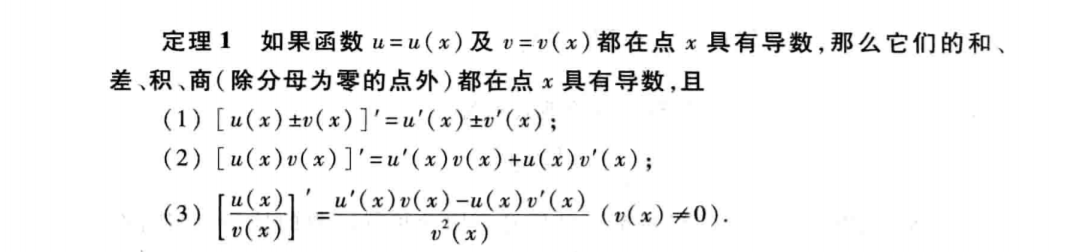1.2、证明，通过极限证明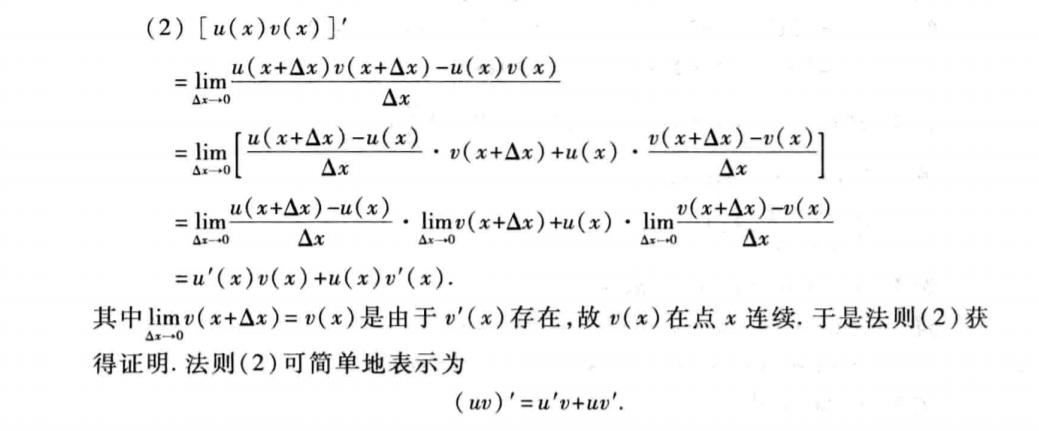二、反函数的求导法则
2.1、定理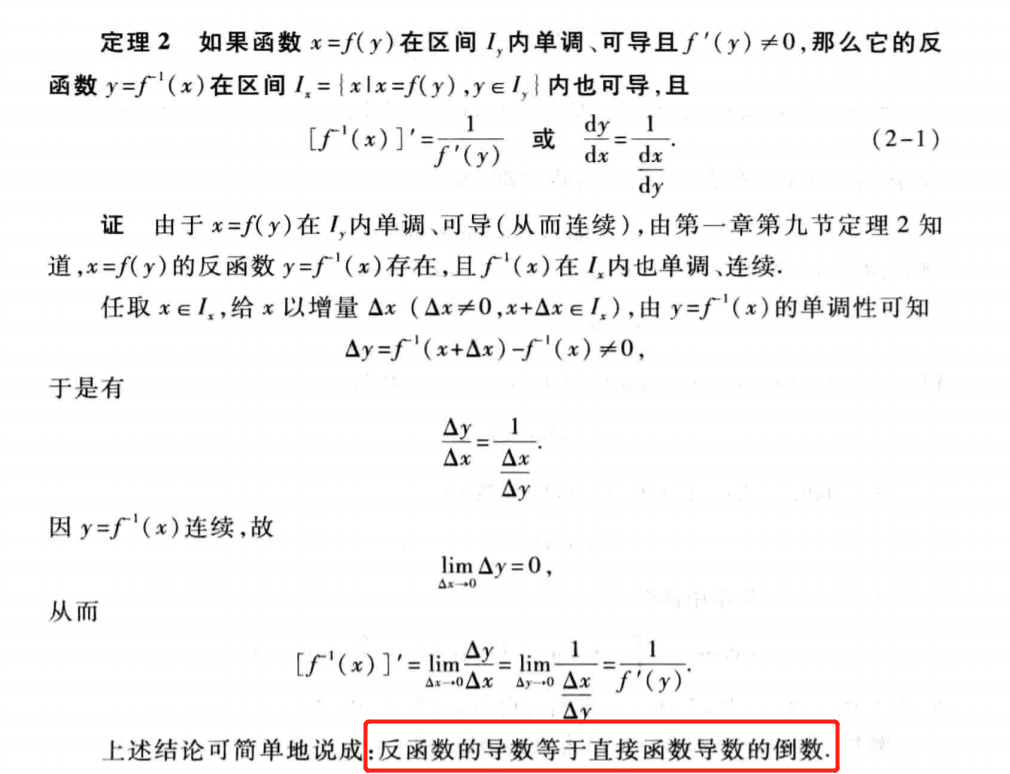2.2、证明样例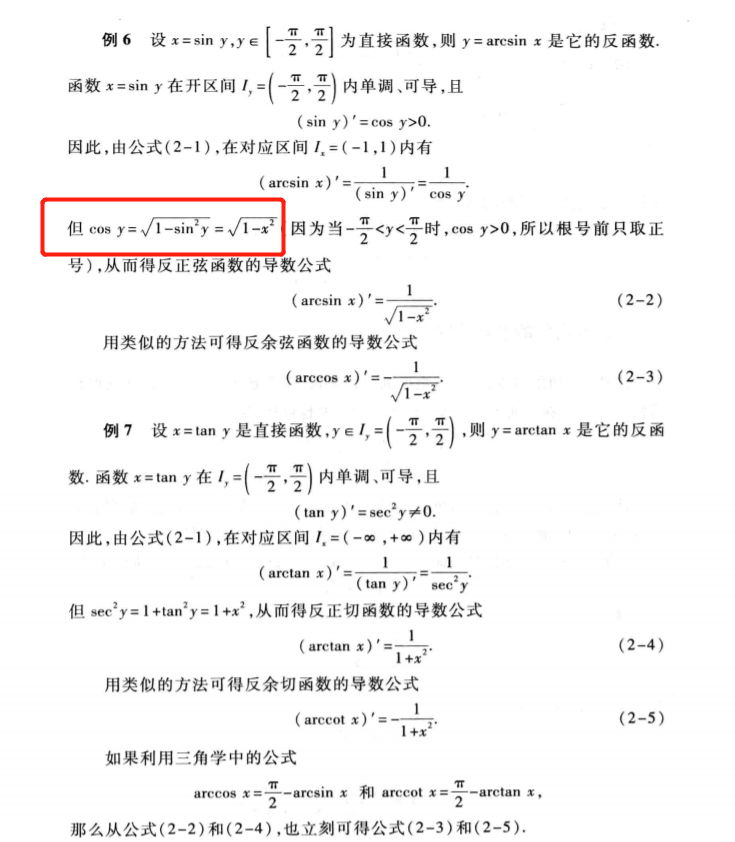三、复合函数的求导法则
3.1、定理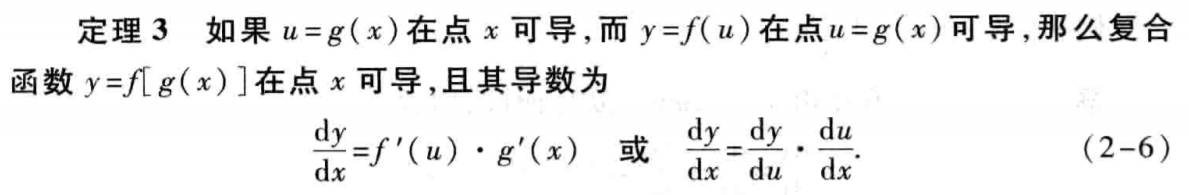3.2、证明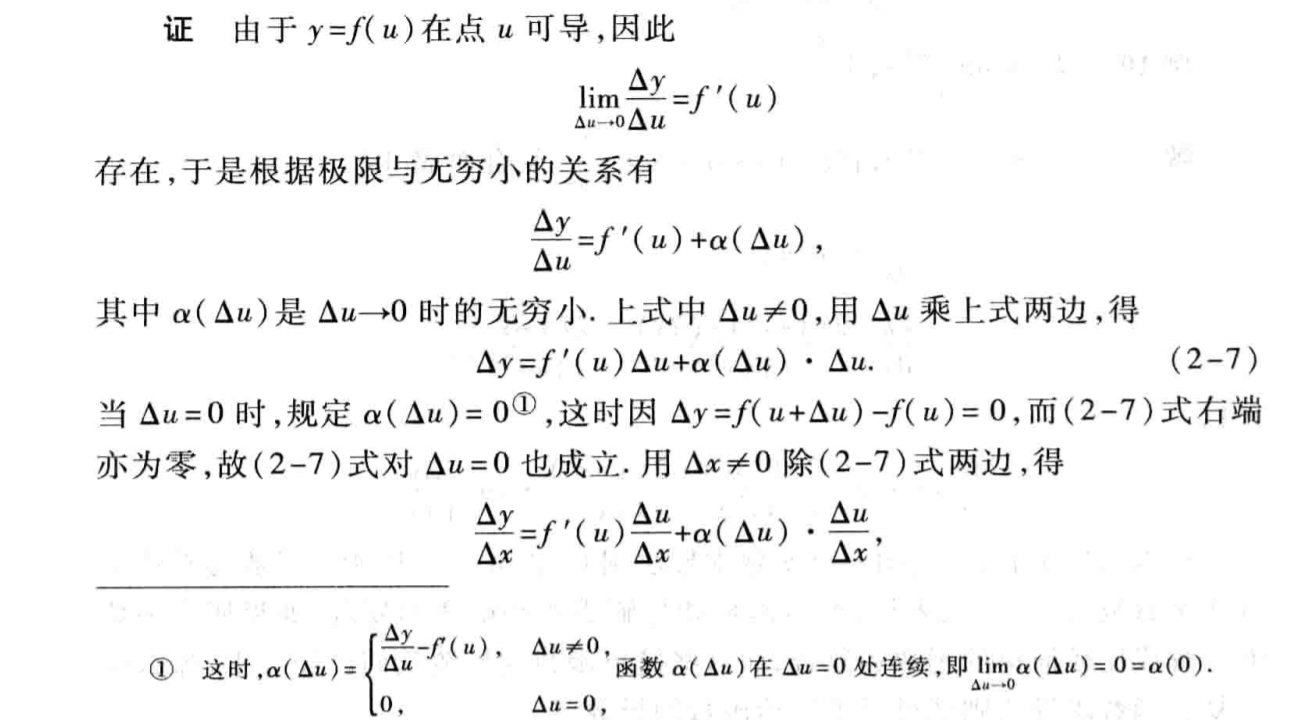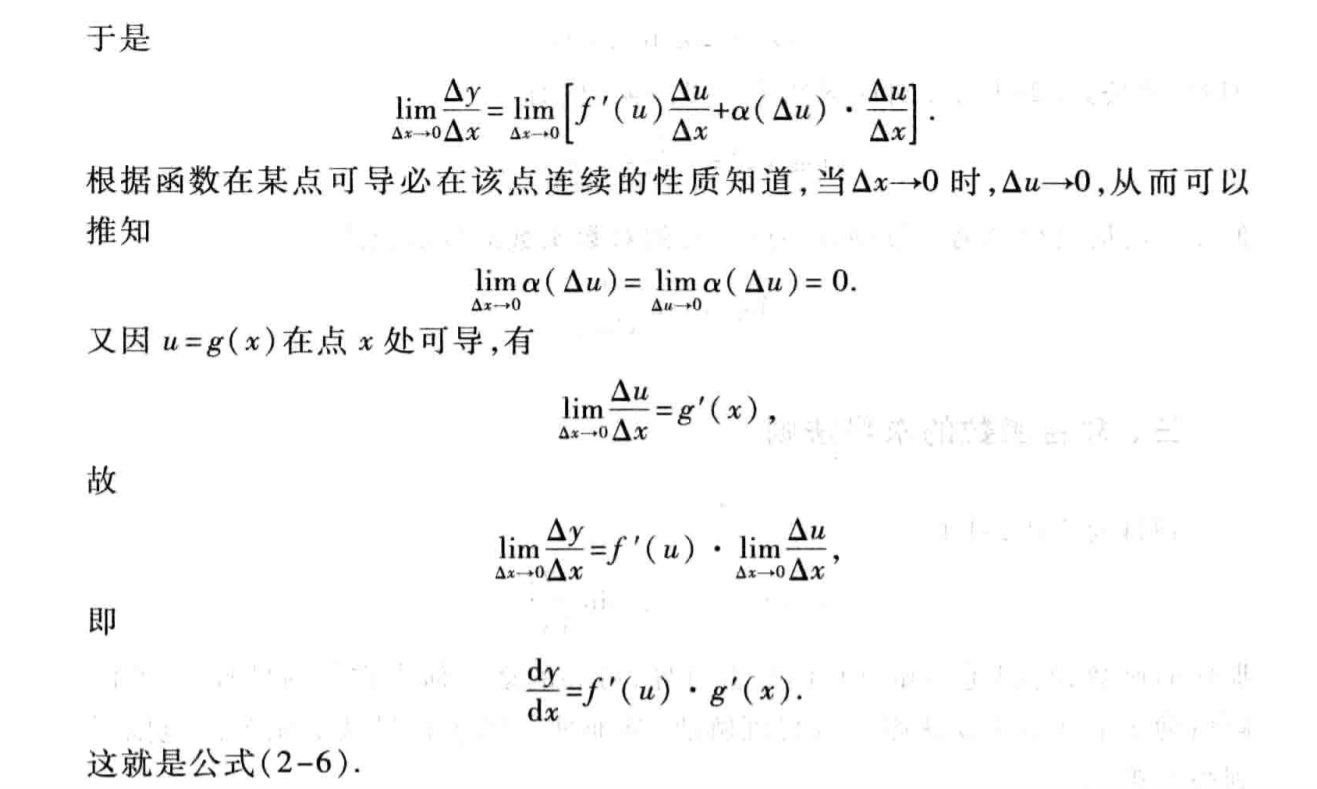3.3、样例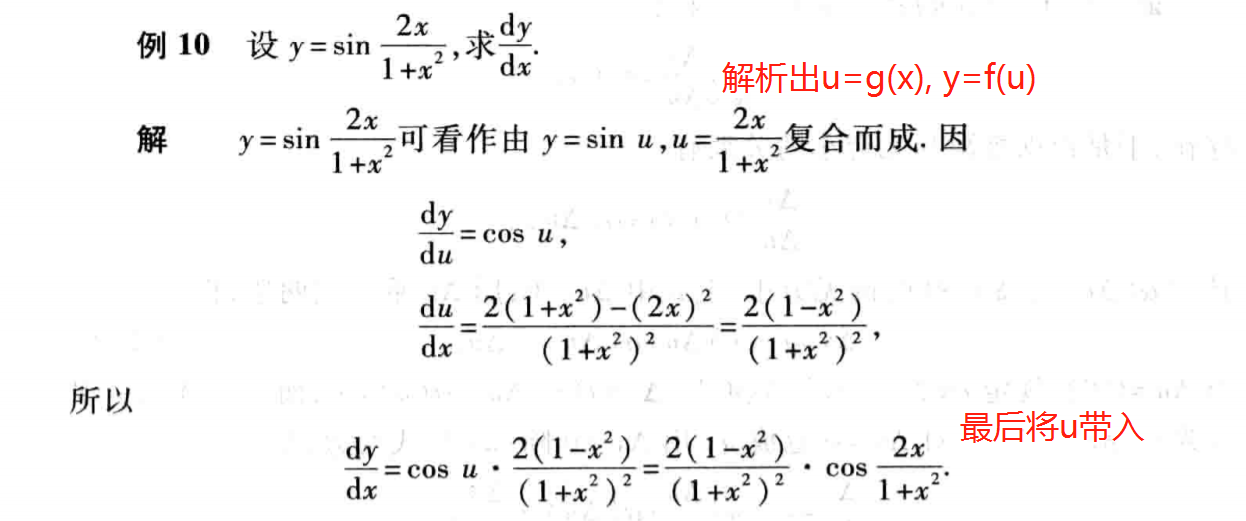3.4、推广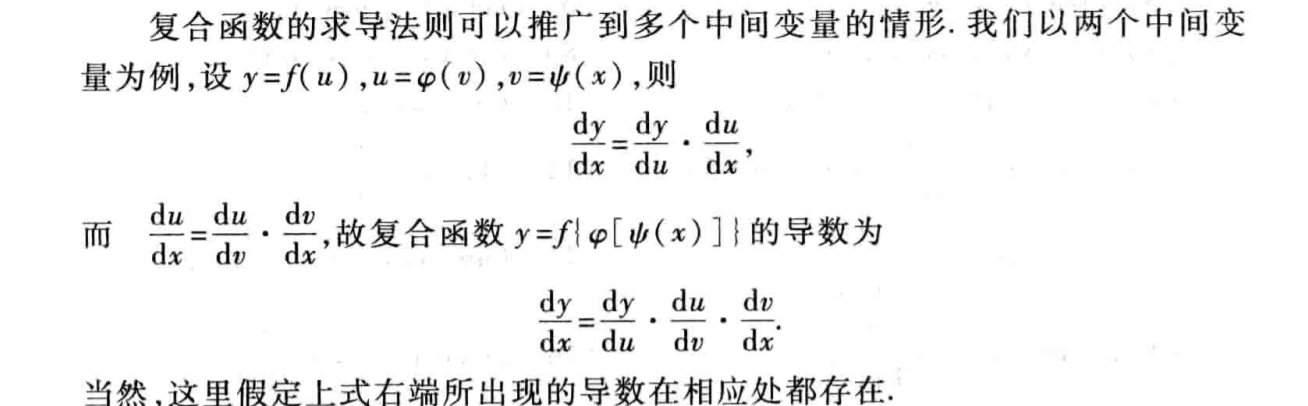3.4.1、样例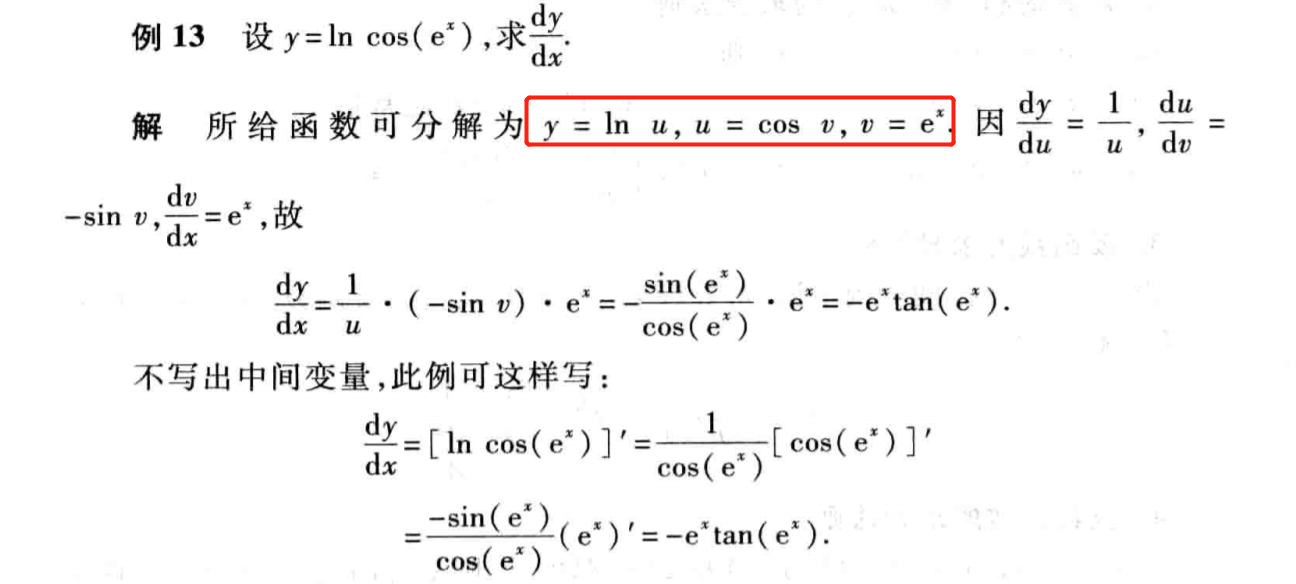展开全文• 该文档总结了矩阵对矩阵、矩阵对向量、向量对矩阵、向量对向量、元素对矩阵、元素对向量的求导法则，非常有用！
• 矩阵、向量求导法则 中文版 包含所有情况和公式，适合翻阅查找 英文版包含推导，非常详细，适合精度，包含了矩阵tr技巧
• ## 链式求导法则

万次阅读 2018-05-30 13:46:16
链式法则是微积分中的求导法则，用以求一个复合函数的导数。所谓的复合函数，是指以一个函数作为另一个函数的自变量。如f(x)=3x，g(x)=x+3，g(f(x))就是一个复合函数，并且g(f(x))=3x+3链式法则(chain rule)：若h(x)...
• ## 矩阵和向量的求导法则

千次阅读 多人点赞 2018-12-16 20:46:51
在Machine Learning 和Deep Learning里面 经常涉及对矩阵和向量的求导，本文介绍一下常用的求导法则
• 某乎有总结的很好的矩阵求导法则叫“矩阵求导术”：https://zhuanlan.zhihu.com/p/24709748https://zhuanlan.zhihu.com/p/24863977迹技巧(trace trick)：标量套上迹：转置：。线性：。矩阵乘法交换：，其中与尺寸...矩阵
• 1. 函数的和，差，积，商的求导法则 2. 反函数求导法则 3. 复合函数的求导法则 4. 例子： 转载于:https://www.cnblogs.com/dreamnk/archive/2011/12/05/2285557.html...
• BP算法的文章很多，但是详解BP算法中的链式求导法则应该只此一家了。包括Hinton关于BP网络的原始论文，对链式求导法则也只是一带而过。 文章先从简化版本的链式法则讲起，再将其应用到BP算法中。 简化版本的链式法则...机器学习 神经网络 深度学习
• 数学 - 基本初等函数导数公式及求导法则 三角函数相关运算 指数和对数函数相关运算 对数函数的强大之处在于可以变积为和，变商为差，化幂为系数。在求幂指函数或某些复杂表达式的函数的导数时，将原来的函数...
• 多元复合函数的求导法则 注：复合函数为向量值函数。
• 微分1、导数1.1 例题—导数定义求导(important)1.2 单侧导数1.3 例题—判断是否可导2、函数的求导法则2.1 定理一 线性组合求导的传递性2.2 定理二 反函数的求导法则2.2.1 例题—利用反函数求导法则求导2.3 定理三 ...
• 【数学】 隐函数求导法则 本篇内容我们说一下隐函数求导的法则，之前在初次接触导数的时候，我们有总结过一部分隐函数求导的内容，虽然和本篇的内容有一部分相似，但是可以再看一看用于对比理解。上正文。 一、......